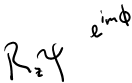Hydrogen part II

L = 0 there is only one state since it is a sphere

l= 1 there are three states but it depends on the orientation (superposition of 3 states)

L = 2 there are 5 states that get superimposed

L = (2l + 1

You should make it complex

x=rsin#cos\$

y=rsin#cos\$

z= rcos#Group- things that you can do, combined you get a rotation (5 + 10 = 15 degrees)

Rotations don’t commute

One rotation times the second isnt always the same depending on the order

1925.                  Electron spin

Found the 2 state

Can be spin up or spin down

If it is spin up, then there is a probability for it to be spin up or spin down

Pauli would rock on his chair so the experiments don’t work

The Pauli affect

1926.                  Wave functions

L = 0 and spin 1/2

(1/2 because you want to get the even numbers for l)

Electron in orbit has a magnetic moment proportional to l and the spin

So its related to the angular momentum

Classical vs. quantum for angular momentum

L = 0 X spin 1/2  = 1/2

L = 1 X spin 1/2 = 6 states but only two of them linearly combine

= split into 2 states and four states

1/2 + 3/2

L = 2 X spin 1/2 = 3/2 + 5/2

1932.                  Neutron was found

1936.                  Nuclear fission

They didn’t go any farther in atomic level because we had the Dirac theory

But anytime you squared the field it would be infinity

It was found through experiment that 2s 1/2 was less energy than 2p 1/2

They are separated by 1057 MHz and this is called the Lamb shift

This went against Dirac's theory that they were equal

1947.                  Relativistic equations were made in America but also in Japan

21 cm line

The proton also spins

Learn about structure of galaxy

So the electron can be spin up or down

The proton can be spin up or down too